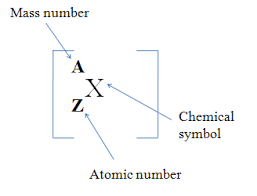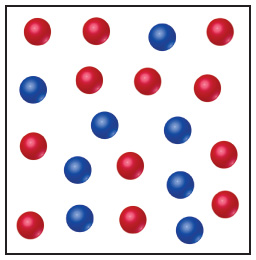# Problem: The following diagram is a representation of 20 atoms of a fictitious element, which we will call nevadium (Nv). The red spheres are 293Nv, and the blue spheres are 295Nv.If the mass of 293Nv is 293.15 amu and that of 295Nv is 295.35 amu , what is the atomic weight of Nv?

###### FREE Expert Solution

Isotopes are atoms with the same number of protons, but different numbers of neutronsThe steps that have to be followed to find the atomic mass or average mass of the element are as follows:

Step 1: Count the number of atoms in both types of atoms present in Nevadium.

Red atoms: 293Nv = 12 atoms

Blue atoms: 295Nv = 8 atoms

98% (206 ratings)###### Problem Details

The following diagram is a representation of 20 atoms of a fictitious element, which we will call nevadium (Nv). The red spheres are 293Nv, and the blue spheres are 295Nv.

If the mass of 293Nv is 293.15 amu and that of 295Nv is 295.35 amu , what is the atomic weight of Nv?